Smartick is an advanced online program that teaches kids math and coding in only 15 min. a day

May25

# Learn Multiplication Algorithm Using Blocks

Blocks are extremely useful for learning mathematics, and operations in particular. Therefore, this week we’re going to learn the multiplication algorithm using blocks to help us.

Do you already know how to multiply by one digit? If the answer is NO, you’ll learn the most simple way to multiply and understand the algorithm in this post. If your answer is YES, then look for the method that you’ve learned.

Let’s get started!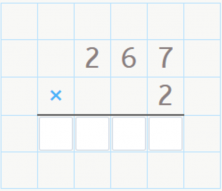We’re going to do the multiplication problem 267 x 2. The first thing we need to do is represent the number 267 with the blocks.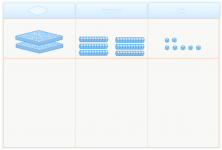As you can see, the number 267 is made up of 2 hundreds, 6 tens, and 7 units.

Now we can start multiplying the units by 2! 7 x 2 = 14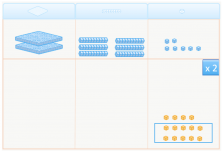As you already know, a group of 10 units makes a rod of ten. So, we select 10 units to move into the tens column.

Now we multiply the tens: 6 x 2 = 12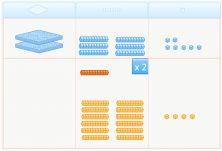Again you know that a group of 10 tens makes a flat of one-hundred, so we select 10 tens to move to the hundreds column.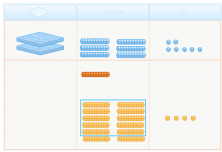Now we multiply the hundreds: 2 x 2 = 4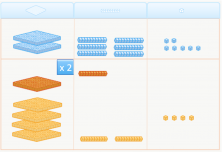We’ve finished the multiplication! There are 5 hundreds, 3 tens, and 4 units. Therefore, the result of the multiplication is 534.

What do you think? Now we’re going to see how the method works for normal vertical multiplication.

• We multiply the units:

7 x 2 = 14. We write down the 4 in the units column and we carry the 1 (the 1 ten that we’ve made).

• We multiply the tens:

6 x 2 = 12. We add the 1 that we carried and we get 13. Then we write down the 3 in the tens column and we carry the 1 (the 1 hundred that we’ve made).

• We multiply the hundreds:

2 x 2 = 4. We add the 1 that we carried and we get 5.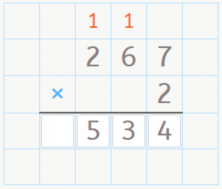Did you like that? Did you find it useful? Share this post with your friends and classmates so they can learn the multiplication algorithm using blocks too!